http://en.wikipedia.org/wiki/Beta_distribution

The general from of the function is:

Between a AND b with coefficient c and d

y = ((x-a)^(c-1) (b-x)^(d-1))/(b-a)^(c+d-1)

y'=(x-a)^(c-2) (b-x)^(d-2) (b-a)^(-c-d+1) (a (d-1)+b (c-1)-x (c+d-2))y'=(x-a)^(c-1) (b-x)^(d-1) (b-a)^(-c-d+1) (a (d-1)+b (c-1)-x (c+d-2))/((x-a)(b-x))therefore the differential equation is: y’=y (a (d-1)+b (c-1)-x (c+d-2))/((x-a)(b-x))if a=0 and b=1 it is a solution to

is a solution to this differential equation

=================================================================================
To make sure that the area below y is one and convert it to a probability density function we find the
integral_a^b (x-a)^(c-1) (b-x)^(d-1) (b-a)^(-c-d+1) dx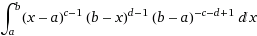which we cal B(c,d) then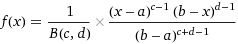f(x)=(1/((gamma(c)*gamma(d))/Gamma(c+d)))*((x-a)^(c-1) (b-x)^(d-1))/(b-a)^(c+d-1)is a probability density function with an area of 1.

========================================================================When and  are not equal the curve doesn’t approach 0 asymptotically at that end but has a peak closer to that end.

This means if is smaller then the peak will be close to a.

Beta curve with =1 is =3  b=1 f=1/B*(x-a)^0(b-x)^2

f=1/B*(x-a)^0(5-x)^2

f=(5-x)^2

is a derivative of:

f'(x)=2(x-5)

————————————————————————

The following is similar to y=e^-x

Exponential curve which will  be 0 at infinity and is a solution to: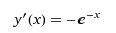When power of positive x is less than 1 and less than the power negative x the curve is monotonous decreasing.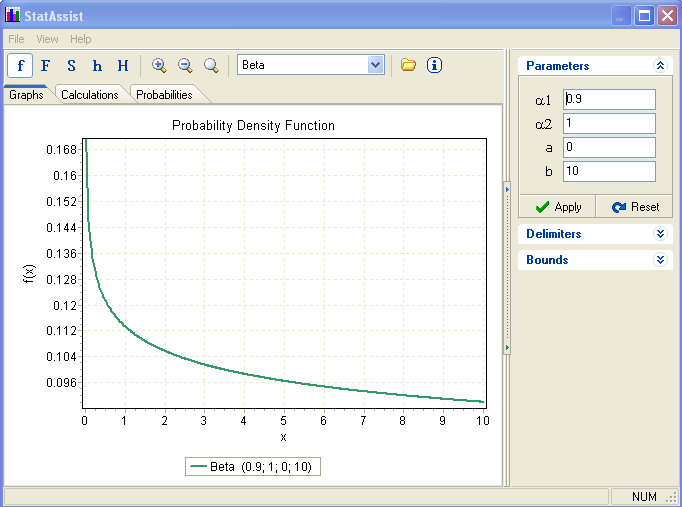When power of positive x is less than the power negative x the curve is skewed to right

If 1 < α < β then mode ≤ median ≤ mean. Expressing the mode (only for α, β > 1), and the mean in terms of α and β:$\frac{ \alpha - 1 }{ \alpha + \beta - 2 } \le \text{median} \le \frac{ \alpha }{ \alpha + \beta } ,$$\text{median} \approx \frac{\alpha - \tfrac{1}{3}}{\alpha + \beta - \tfrac{2}{3}} \text{ for } \alpha, \beta \ge 1.$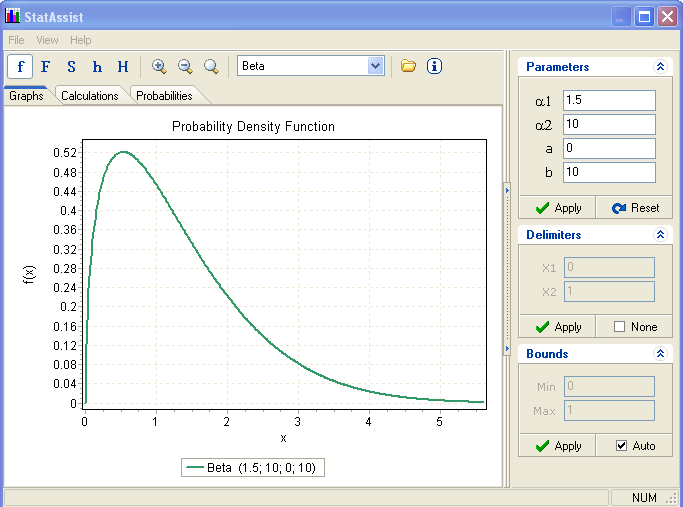——————————In general with$\text{median} \approx \frac{\alpha - \tfrac{1}{3}}{\alpha + \beta - \tfrac{2}{3}} \text{ for } \alpha, \beta \ge 1.$=2 is$\text{median} \approx \frac{\alpha - \tfrac{1}{3}}{\alpha + \beta - \tfrac{2}{3}} \text{ for } \alpha, \beta \ge 1.$=3

y=((x-a)(b-x)^2)

is a solution towith$\text{median} \approx \frac{\alpha - \tfrac{1}{3}}{\alpha + \beta - \tfrac{2}{3}} \text{ for } \alpha, \beta \ge 1.$=2 is$\text{median} \approx \frac{\alpha - \tfrac{1}{3}}{\alpha + \beta - \tfrac{2}{3}} \text{ for } \alpha, \beta \ge 1.$=3 a=0 b=5

y=(x(5-x)^2)

is a solution to:In general with$\text{median} \approx \frac{\alpha - \tfrac{1}{3}}{\alpha + \beta - \tfrac{2}{3}} \text{ for } \alpha, \beta \ge 1.$=2 is$\text{median} \approx \frac{\alpha - \tfrac{1}{3}}{\alpha + \beta - \tfrac{2}{3}} \text{ for } \alpha, \beta \ge 1.$=3 and a=0

y=((x)(b-x)^2)

is a solution to:In general with$\text{median} \approx \frac{\alpha - \tfrac{1}{3}}{\alpha + \beta - \tfrac{2}{3}} \text{ for } \alpha, \beta \ge 1.$=3 is$\text{median} \approx \frac{\alpha - \tfrac{1}{3}}{\alpha + \beta - \tfrac{2}{3}} \text{ for } \alpha, \beta \ge 1.$=2 and a=0

y=((x)^2(b-x))

is a solution to: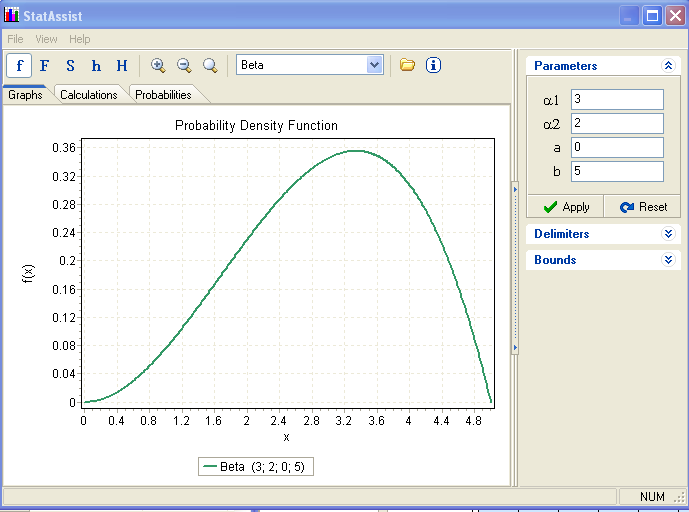In general with$\text{median} \approx \frac{\alpha - \tfrac{1}{3}}{\alpha + \beta - \tfrac{2}{3}} \text{ for } \alpha, \beta \ge 1.$=3 is$\text{median} \approx \frac{\alpha - \tfrac{1}{3}}{\alpha + \beta - \tfrac{2}{3}} \text{ for } \alpha, \beta \ge 1.$=3

y=((x-a)^2(b-x)^2)

is a solution toThis will have a peak at (a+b)/2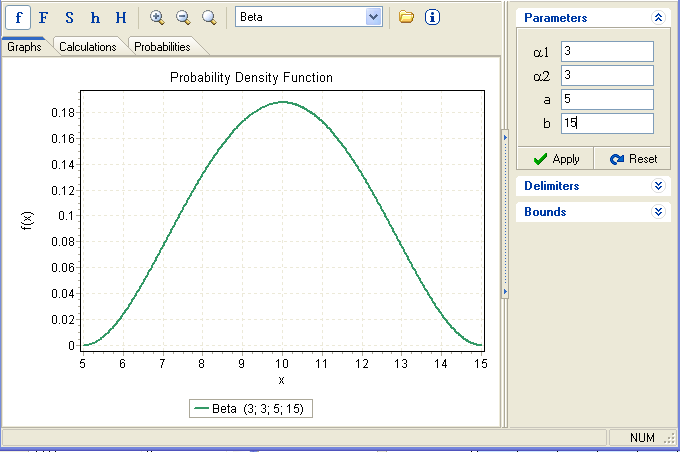In general with$\text{median} \approx \frac{\alpha - \tfrac{1}{3}}{\alpha + \beta - \tfrac{2}{3}} \text{ for } \alpha, \beta \ge 1.$=5 is$\text{median} \approx \frac{\alpha - \tfrac{1}{3}}{\alpha + \beta - \tfrac{2}{3}} \text{ for } \alpha, \beta \ge 1.$=5

y=((x-a)^4(b-x)^4)

is a solution toThis will have a peak at (a+b)/2

the mean is$\text{median} \approx \frac{\alpha - \tfrac{1}{3}}{\alpha + \beta - \tfrac{2}{3}} \text{ for } \alpha, \beta \ge 1.$$\text{median} \approx \frac{\alpha - \tfrac{1}{3}}{\alpha + \beta - \tfrac{2}{3}} \text{ for } \alpha, \beta \ge 1.$

This dynamic will be dictated by the distance from (a+b)/2, the curve will have a peak at (a+b)/2 and y will asymptotically reach 0 at x=a and x=b.

Beta distribution with$\text{median} \approx \frac{\alpha - \tfrac{1}{3}}{\alpha + \beta - \tfrac{2}{3}} \text{ for } \alpha, \beta \ge 1.$=$\text{median} \approx \frac{\alpha - \tfrac{1}{3}}{\alpha + \beta - \tfrac{2}{3}} \text{ for } \alpha, \beta \ge 1.$=3 is bell shaped and$\text{median} \approx \frac{\alpha - \tfrac{1}{3}}{\alpha + \beta - \tfrac{2}{3}} \text{ for } \alpha, \beta \ge 1.$=$\text{median} \approx \frac{\alpha - \tfrac{1}{3}}{\alpha + \beta - \tfrac{2}{3}} \text{ for } \alpha, \beta \ge 1.$=5 is very similar to normal it always has 0 skewness and kurtosis will approach 0 when$\text{median} \approx \frac{\alpha - \tfrac{1}{3}}{\alpha + \beta - \tfrac{2}{3}} \text{ for } \alpha, \beta \ge 1.$=$\text{median} \approx \frac{\alpha - \tfrac{1}{3}}{\alpha + \beta - \tfrac{2}{3}} \text{ for } \alpha, \beta \ge 1.$=infinity 🙂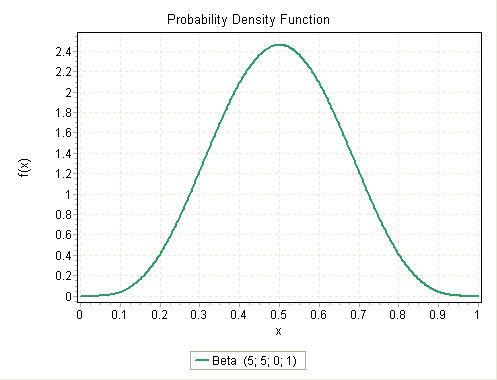==========================================================

==========================================================

Below is the evolution of Beta as shape parameters change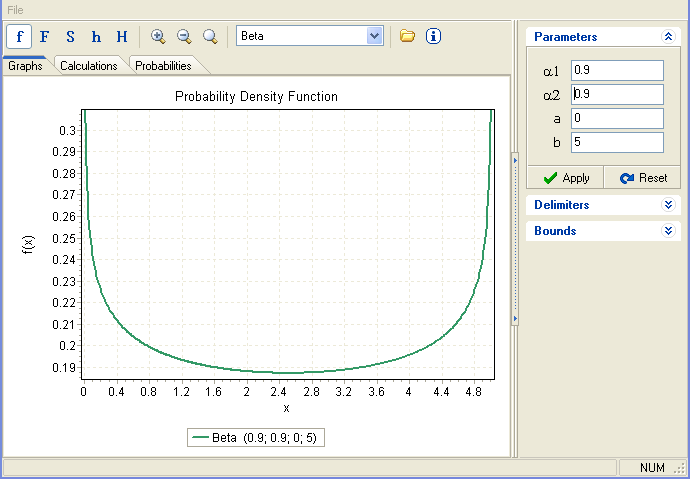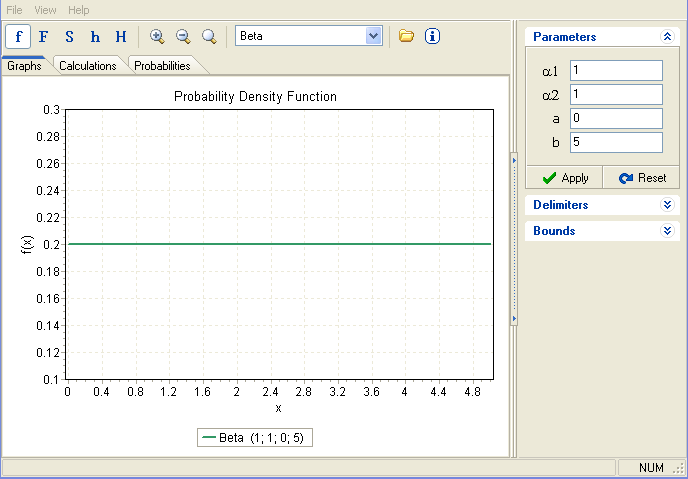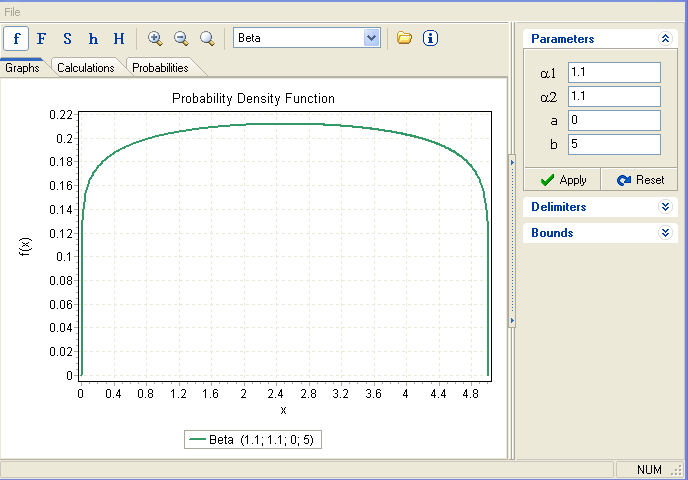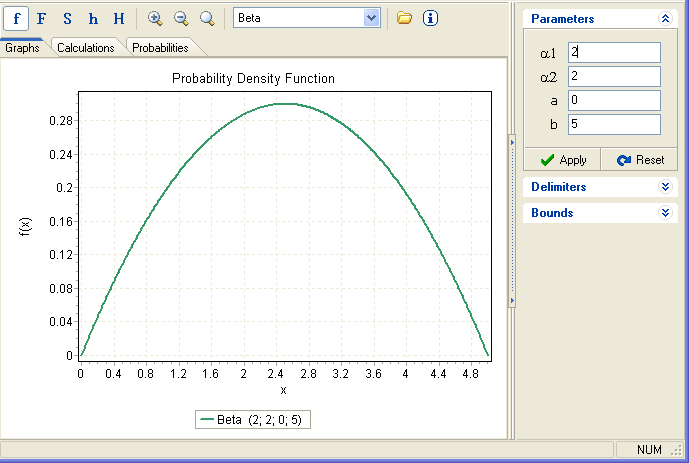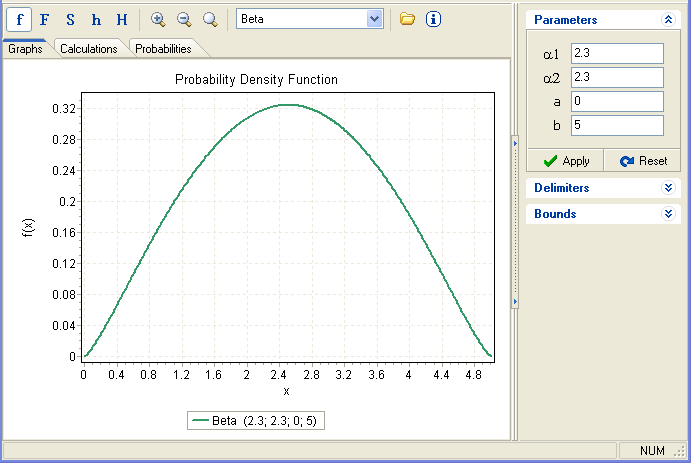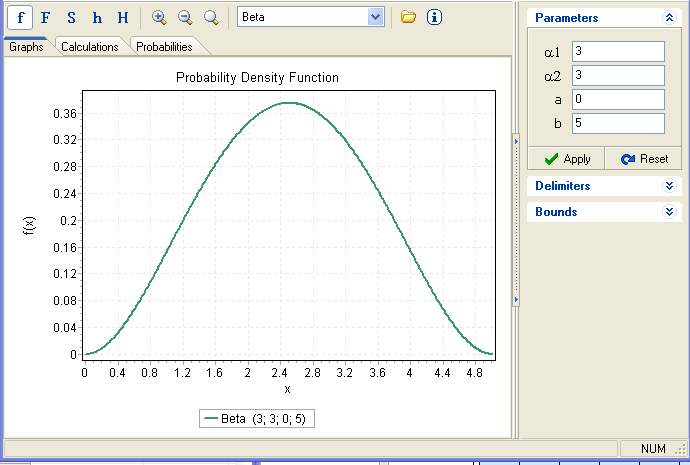Since 11 April 2023: 322 total views,  1 views today